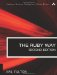# Section 5.9. Working with Rational Values

### 5.8. Using BigDecimal

The bigdecimal standard library enables us to work with large numbers of significant digits in fractional numbers. In effect, it stores numbers as arrays of digits rather than converting to a binary floating point representation. This allows arbitrary precision, though of course at the cost of speed.

To motivate ourselves, look at the following simple piece of code using floating point numbers:

`if (3.2 - 2.0) == 1.2   puts "equal" else   puts "not equal"     # prints "not equal"! end`

This is the sort of situation that BigDecimal helps with. However, note that with infinitely repeating decimals, we still will have problems. For yet another approach, see the upcoming section 5.9 "Working with Rational Values."

A BigDecimal is initialized with a string. (A Float would not suffice because the error would creep in before we could construct the BigDecimal object.) The method BigDecimal is equivalent to BigDecimal.new; this is another special case where a method name starts with a capital letter. The usual mathematical operations such as + and * are supported. Note that the to_s method can take a parameter to specify its format. For more details, see the ruby-doc.org site.

`require 'bigdecimal' x = BigDecimal("3.2") y = BigDecimal("2.0") z = BigDecimal("1.2") if (x - y) == z   puts "equal"         # prints "equal"! else   puts "not equal" end a = x*y*z a.to_s                 # "0.768E1" (default: engineering notation) a.to_s("F")            # "7.68"    (ordinary floating point)`

We can specify the number of significant digits if we want. The precs method retrieves this information as an array of two numbers: the number of bytes used and the maximum number of significant digits.

`x = BigDecimal("1.234",10) y = BigDecimal("1.234",15) x.precs                      # [8, 16] y.precs                      # [8, 20]`

The bytes currently used may be less than the maximum. The maximum may also be greater than what you requested (because BigDecimal TRies to optimize its internal storage).

The common operations (addition, subtraction, multiplication, and division) have counterparts that take a number of digits as an extra parameter. If the resulting significant digits are more than that parameter specifies, the result will be rounded to that number of digits.

`a = BigDecimal("1.23456") b = BigDecimal("2.45678") # In these comments, "BigDecimal:objectid" is omitted c  = a+b         # <'0.369134E1',12(20)> c2 = a.add(b,4)  # <'0.3691E1',8(20)> d  = a-b         # <'-0.122222E1',12(20)> d2 = a.sub(b,4)  # <'-0.1222E1',8(20)> e  = a*b         # <'0.3033042316 8E1',16(36)> e2 = a.mult(b,4) # <'0.3033E1',8(36)> f  = a/b         # <'0.5025114173 8372992290 7221E0',24(32)> f2 = a.div(b,4)  # <'0.5025E0',4(16)>`

The BigDecimal class defines many other functions such as floor, abs, and others. There are operators such as % and ** as you would expect, along with relational operators such as <). The == is not intelligent enough to round off its operands; that is still the programmer's responsibility.

The BigMath module defines constants E and PI to arbitrary precision. (They are really methods, not constants.) It also defines functions such as sin, cos, exp, and others, all taking a digits parameter.

The following sublibraries are all made to work with BigDecimal.

 bigdecimal/math The BigMath module bigdecimal/jacobian Methods for finding a Jacobian matrix bigdecimal/ludcmp The LUSolve module, for LU decomposition bigdecimal/newton Provides nlsolve and norm

These sublibraries are not documented in this chapter. For more information, consult the ruby-doc.org site or any detailed reference.The Ruby Way, Second Edition: Solutions and Techniques in Ruby Programming (2nd Edition)
ISBN: 0672328844
EAN: 2147483647
Year: 2004
Pages: 269
Authors: Hal Fulton

Similar book on Amazon